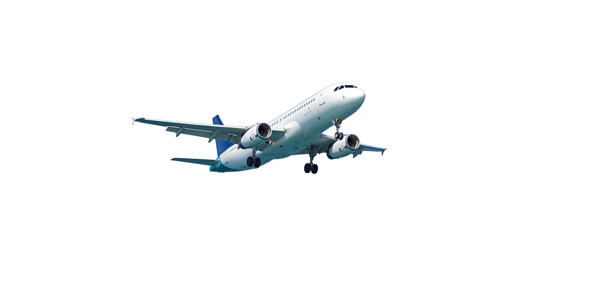# Can You Pass This Aircraft Electrical System Test?

100 Questions | Attempts: 984
ShareSettingsAir transport is the fastest means of transportation, and it does not come with some dangers too. Being an electrical engineer is one thing, but being an aircraft electrical system engineer is another. This job requires more knowledge but picks some from the basics. These are the same questions you will see in the CBT, so try to know the answers as well. For the Multiple Choice, try to understand the answer rather than memorize it.

• 1.
The polar axis is the
• A.

Circle equal distance from the poles

• B.

Axis about which the earth rotates

• C.

Circle formed by any plane that passes through the center of the earth

• D.

Axis about which the moon rotates

• 2.
What is formed on the surface of the Earth by any plane that passes through the center of the Earth and divides it into two equal parts?
• A.

Parallel of latitude

• B.

Great circle

• C.

Meridian of longitude

• D.

Equator

• 3.
Which system utilizes the equator as one fixed regerence point and the Greenwich meridian as the other fixed reference point?
• A.

Instrument landing

• B.

Aircraft landing

• C.

Geographic coordinate

• D.

• 4.
The prime meridian is the
• A.

Circle equal distance between the north and south poles

• B.

Meridian passing through Greenwich, England

• C.

Meridian designated the 180 degree meridian

• D.

Circle designated 0 degree latitude

• 5.
From the prime meridian east to the international dateline, meridians are assigned values of
• A.

0 to 90 degrees east

• B.

0 to 180 degrees west

• C.

0 to 90 degrees west

• D.

0 to 180 east

• 6.
From the prime meridian west to the international dateline, meridians are assigned values of
• A.

0 to 90 degrees east

• B.

0 to 90 degrees west

• C.

0 to 180 degrees west

• D.

0 to 180 degrees east

• 7.
In navigation, the primary reference is
• A.

90 degrees

• B.

North

• C.

The equator

• D.

0 degrees

• 8.
North on a compass rose is
• A.

90 degrees

• B.

270 degrees

• C.

180 degrees

• D.

0 degrees

• 9.
Relative bearing of an aircraft is the direction measured clockwise from
• A.

The aircraft heading to the object

• B.

True north to the object

• C.

0 degrees to the object

• D.

The object to true north

• 10.
The effect of wind on an aircraft is
• A.

Unimportant

• B.

Negligible

• C.

Significant

• D.

Slight

• 11.
The sideward displacement of an aircraft by the wind is called
• A.

Deviation

• B.

Yaw

• C.

Groundspeed

• D.

Drift

• 12.
The rate of movement of an aircraft relative to the Earth's surface is called
• A.

Airspeed

• B.

Velocity

• C.

Groundspeed

• D.

Drift

• 13.
What is used to correct true heading and allow the aircraft to make good the desired true course?
• A.

Drift correction

• B.

Ground speed correction

• C.

Airspeed correction

• D.

Wind disbursement

• 14.
A vector diagram that shows the effect of the wind on the flight of an aircraft is called the
• A.

Wind triangle

• B.

• C.

Destination chart

• D.

Flight path

• 15.
On navigation charts, the lines that connect points of equal magnetic variation are called
• A.

Deviation lines

• B.

Agonic

• C.

Variation lines

• D.

Isogonic

• 16.
If the airspeed of the aircraft is too fast, a falling bomb
• A.

Hits the target

• B.

Overshoots the target

• C.

Falls to the side of the target

• D.

Falls short of the target

• 17.
Air resistance causing a falling bomb to
• A.

Fall unpredictably

• B.

Lag

• C.

• D.

Fall rapid

• 18.
A head wind causes a falling bomb to
• A.

Fall short of the target

• B.

Fall left or right of the target

• C.

Hit the target

• D.

Overshoot the target

• 19.
A cross wind causes a falling bomb to
• A.

Hit the target

• B.

Fall short of the target

• C.

Fall left or right of the target

• D.

Overshoot the target

• 20.
The configuration of the onboard weapons can be described in the
• A.

Release table

• B.

Stores table

• C.

Gravity table

• D.

Action point table

• 21.
The preplanned flight route can be described in the
• A.

Stores table

• B.

Gravity table

• C.

Release table

• D.

Action point table

• 22.
Which is not a basic inertial navigation system component?
• A.

Indicator

• B.

Computer

• C.

Integrator

• D.

Accelerometer

• 23.
The changing of position with respect to some other body is called
• A.

Speed

• B.

Reference

• C.

Motion

• D.

Velocity

• 24.
Which is used to maintain accelerometer orientation in the inertial navigation system?
• A.

Platform

• B.

Summing unit

• C.

Integrator

• D.

Computer

• 25.
What LRU consists of a gyro-stabilized platform, platform electronics, navigation computer, input/output functions, and power supply?
• A.

• B.

• C.

Terrain-following avionics control unit

• D.

Doppler velocity sensor

## Related TopicsBack to top
×

Wait!
Here's an interesting quiz for you.